Next: Quadratic Stark Effect Up: Time-Independent Perturbation Theory Previous: Two-State System

# Non-Degenerate Perturbation Theory

Let us now generalize our perturbation analysis to deal with systems possessing more than two energy eigenstates. The energy eigenstates of the unperturbed Hamiltonian,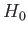, are denoted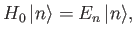(7.19)

where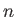runs from 1 to. The eigenketsare orthonormal, and form a complete set. Let us now try to solve the energy eigenvalue problem for the perturbed Hamiltonian: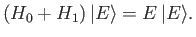(7.20)

We can expressas a linear superposition of the unperturbed energy eigenkets,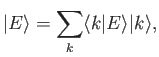(7.21)

where the summation is from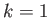to. Substituting the previous equation into Equation (7.20), and right-multiplying by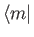, we obtain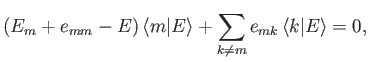(7.22)

where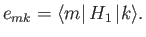(7.23)

Let us now develop our perturbation expansion. We assume that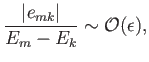(7.24)

for all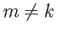, where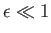is our expansion parameter. We also assume that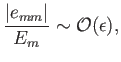(7.25)

for all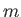. Let us search for a modified version of theth unperturbed energy eigenstate for which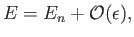(7.26)

and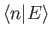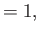(7.27)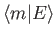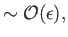(7.28)

for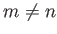. Suppose that we write out Equation (7.22) for, neglecting terms that are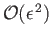according to our expansion scheme. We find that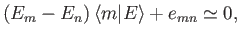(7.29)

giving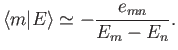(7.30)

Substituting the previous expression into Equation (7.22), evaluated for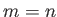, and neglecting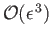terms, we obtain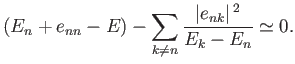(7.31)

Thus, the modifiedth energy eigenstate possesses the eigenvalue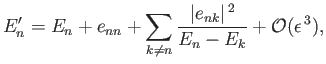(7.32)

and the eigenket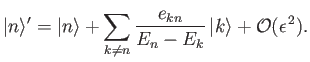(7.33)

Note that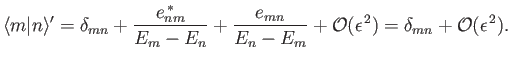(7.34)

Thus, the modified eigenkets remain orthonormal to.

Note, finally, that if the perturbing Hamiltonian,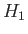, commutes with the unperturbed Hamiltonian,, then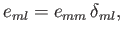(7.35)

and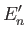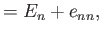(7.36)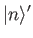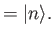(7.37)

The previous two equations are exact (i.e., they hold to all orders in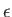).Next: Quadratic Stark Effect Up: Time-Independent Perturbation Theory Previous: Two-State System
Richard Fitzpatrick 2016-01-22